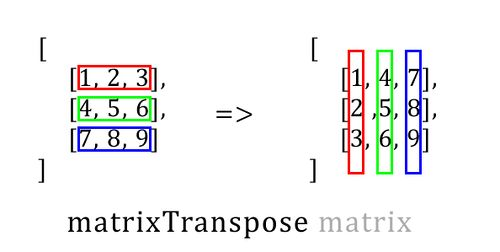# matrixTranspose

Click on the images for descriptions

Game:
Arma 3
Version:
1.92

### Description

Description:
Returns transposed version of the given matrix. The matrix is an Array of rows. Each row is an Array of corresponding column values for the row. The minimum number of rows is 1.### Syntax

Syntax:
matrixTranspose matrix
Parameters:
matrix: Array - given matrix
Return Value:
Array - transposed matrix

### Examples

Example 1:
```matrixTranspose [ [1,2,3], [3,1,2], [2,3,1] ]; /* returns [ [1,3,2], [2,1,3], [3,2,1] ] */```
Example 2:
```matrixTranspose [ [1,2,3] ]; /* returns [ , ,  ] */```
Example 3:
```matrixTranspose [ , ,  ]; /* returns [ [1,2,3] ] */```